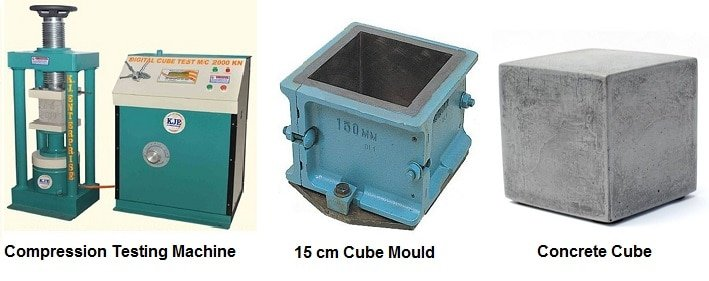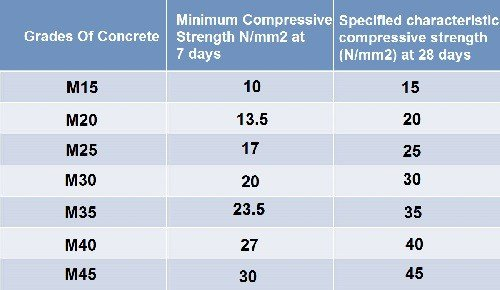# Compressive Strength Test Of Concrete Cubes

Contents

## Compressive Strength Of Concrete Test:

In this article, compressive strength of concrete cube test will be discussed.### Aim:

To determine the compressive strength of concrete cube.

### Apparatus & Equipment:

1. Compression test machine,

2. Cube mould of 15 cm size,

3. Curing Tank,

4. Tamping bar,

5. Weighing balance.

### Specimen For Test:

The cube samples shall be 15 x 15 x 15 cm. If the largest nominal size of the aggregate does not exceed 2 cm, then cube samples of 10 cm may be used as an alternative.

## Casting Of Concrete Cubes For Compressive Strength Test:

### Proportioning:

The ingredients should be proportioned as per design standards.

### Mixing Of Concrete:

The concrete should be mixed either by hand or in a laboratory machine mixer in such a manner as to reduce wastage of water and other materials.

#### Machine Mixing:

The mixing should be done as follows:

1. Add one half of the coarse aggregate into the mixing drum.

2. Then add cement and fine aggregates and finally add the remaining coarse aggregates.

3. Add the required amount of water.

4. Start the mixing machine until the resulting concrete gives a uniform texture.

#### Hand Mixing:

Hand mixing is also adopted  for preparing concrete cubes for compressive strength test. The mixing should be done on a watertight, non-absorbent platform with a trowel or shovel using the following procedure:

1. Mix the cement and fine aggregates thoroughly in dry condition.

2. Add Coarse aggregates and thoroughly mix with cement and fine aggregates.

3. Add the required amount of water and mix it properly until the concrete becomes homogeneous of the desired consistency.

### Sampling Of Concrete Cubes:

1. Fill the mould with freshly mixed concrete in layers approximately 5 cm deep.

2. Compact the concrete either by vibrator or by using the tamping bar. ( Minimum 35 strokes per layer for 15 cm cubes and 25 strokes for 10 cm cubes).

3. Finish the top level of the mould using a trowel.

4. Cover the mould with a glass plate or gunny bag to protect evaporation.

### Curing Of Concrete Cubes For Test:

1. Keep the specimens in a place, free from vibration, in moist air and at a temperature of 27°C ±2° C for 24 hours.

2. After 24 hours, mark and remove the samples from mould.

3. Submerge the cubes immediately in fresh and clean water until taken out prior to the test. The water should be renewed every 7 days.

## Procedure Of Compressive Strength Test :

1. Remove the specimens from the water before 30 minutes of testing.

2. Remove any loose sand or other material from the surface of the specimens and let them dry.

3. Clean the bearing surface of the compression testing machine.

4. Now place the cube in the testing machine in such a manner that the load is applied o the opposite sides of the cubes.

5. Align the axis of the specimen with the centre of thrust of spherically seated platen.

6. Apply the load increasingly at a rate of 140 kg/cm² per minute until the cube collapse.

7. Note down the maximum load applied to the specimen and any other unusual activities at the time of failure.

#### Points To Be Remembered :

Few points must be remembered for compressive strength of concrete testing.

#### 1. Age Of Test:

The test should be done at 7 days and 28 days.

#### 2. Number Of Specimen:

At least three specimens, preferably from different batches for testing at each selected age.

#### Calculation For Compressive Strength Concrete:Let assume the maximum applied load is 400 KN = 400000 N

Cross-sectional area of cube =15 x 15 = 225 cm²

Compressive strength of concrete = 400000/225 = 1778 N/cm² = 1778/9.81 = 181 Kg/cm² [ 1kg =9.81 N]

#### Reports:

 Details Samples Specimen 1 Specimen 2 Specimen 3 Compressive Load (KN) 380 KN 400 KN 425 KN Compressive Strength (Kg/Cm²) (380000/225) / 9.81 = 172 kg/cm² (400000/225) / 9.81 = 181 kg/cm² (425000/225) / 9.81 = 192 kg/cm² Average Compressive Strength = (172+181+192)/3  = 181.66 Kg/cm²

#### Precaution:

This compressive strength test of concrete should be done at a temperature of 27°C ± 2°C.

### Compressive Strength Of Concrete Over Time:### Compressive Strength Of Concrete At 7 & 28 Days:#### Also Read – Slump Test Of Concrete.

Join Telegram Channel – Civil Engineering Daily

### 1 thought on “Compressive Strength Test Of Concrete Cubes”

1. I want COVER BLOCK strange